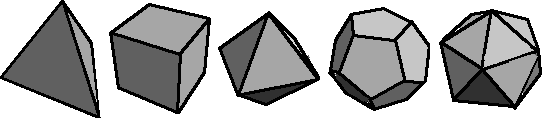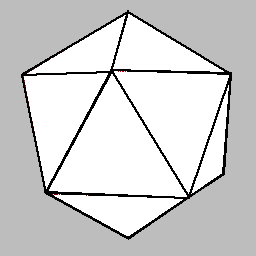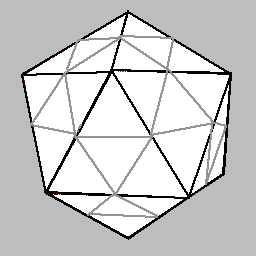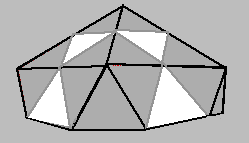## Dome Background

The ancient Greeks developed the area of math known as geometry. Using this branch of math they described five regular solids called the Platonic Solids.

The tetrahedron has 4 sides.
The hexahedron (cube) has 6 sides.
The octahedron has 8 sides.
The dodecahedron has 12 sides.
The icosahedron has 20 sides.Our dome starts with an icosahedron.To make our dome more spherical we divide each triangular face of the icosahedron into 4 triangles.The icosahedron divides very easily in the middle as shown here. Note the new triangles combine to create pentagons (grey), connected  by triangles (white). This hemisphere is made up of 6 pentagons joined with 10 triangles. Each pentagon is constructed with 5 triangles. This structure is still very angular, to make it more "dome like" it is necessary to reshape the structure by moving each "hub" (point where triangles meet) outward.

Page created at: Hila Science Camp: http://hilaroad.com